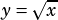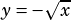On SAT Practice Test 8, I got marked incorrect on Question 7 of the Math No-Calculator section. This is because I said -1 is a valid solution when it isn’t supposed to be. However, if you plug in -1 into the original equation, and say that the square root of 4 is -2, the solution remains true.

So my question is, on the SAT, are you only ever supposed to use the principle square root of numbers? Saying that the square root of 4 = -2 is what rendered my answer incorrect.

Yep, and good you learned this lesson on a practice test and not the real thing! The square root symbol has the properties of a function, which means for any input it only has one output: the positive square root. That’s why when you graphyou don’t get a sideways parabola, you only get half of one (to get the bottom half you have to graph).

Full solution to this question is here, btw.